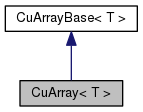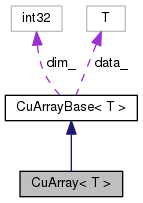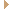CuArray< T > Class Template Reference

Class CuArray represents a vector of an integer or struct of type T. More...

`#include <cu-array.h>`

Inheritance diagram for CuArray< T >:[legend]
Collaboration diagram for CuArray< T >:[legend]

## Public Member Functions

CuArray ()
Default constructor, initialized data_ to NULL and dim_ to 0 via constructor of CuArrayBase. More...

CuArray (MatrixIndexT dim, MatrixResizeType resize_type=kSetZero)
Constructor with memory initialisation. More...

CuArray (const std::vector< T > &src)
Constructor from CPU-based int vector. More...

CuArray (const CuArray< T > &src)
Copy constructor. More...

~CuArray ()
Destructor. More...

void Resize (MatrixIndexT dim, MatrixResizeType resize_type=kSetZero)
Allocate the memory. More...

void Destroy ()
Deallocate the memory and set dim_ and data_ to zero. More...

void CopyFromVec (const std::vector< T > &src)
This function resizes if needed. More...

void CopyFromArray (const CuArrayBase< T > &src)
This function resizes if needed. More...

CuArray< T > & operator= (const CuArray< T > &in)

CuArray< T > & operator= (const std::vector< T > &in)

void Swap (CuArray< T > *other)
Shallow swap with another CuArray<T>. More...

void Read (std::istream &is, bool binary)
I/O. More...

void Write (std::ostream &is, bool binary) constPublic Member Functions inherited from CuArrayBase< T >
MatrixIndexT Dim () const
Return the vector dimension. More...

const T * Data () const
Get raw pointer. More...

T * Data ()

void SetZero ()
Sets the memory for the object to zero, via memset. More...

void CopyFromArray (const CuArrayBase< T > &src)
The caller is responsible to ensure dim is equal between *this and src. More...

void CopyFromVec (const std::vector< T > &src)
The caller is responsible to ensure dim is equal between *this and src. More...

void CopyToVec (std::vector< T > *dst) const
This function resizes *dst if needed. More...

void CopyToHost (T *dst) const
Version of the above function that copies contents to a host array (i.e. More...

void Set (const T &value)
Set to a constant value. More...

void Sequence (const T base)
Fill with the sequence [base ... More...

Min () const
Get minimum value (for now implemented on CPU, reimplement if slow). More...

Max () const
Get minimum value (for now implemented on CPU, reimplement if slow). More...

template<>
void Set (const int32 &value)

template<>
void Sequence (const int32 base)

template<>

template<>
void Sequence (const int32 base)

template<>
void Set (const int32 &value)

template<>Protected Member Functions inherited from CuArrayBase< T >
CuArrayBase ()
Default constructor: make it protected so the user cannot instantiate this class. More...Protected Attributes inherited from CuArrayBase< T >
T * data_
GPU data pointer (if GPU not available, will point to CPU memory). More...

MatrixIndexT dim_
dimension of the vector More...

## Detailed Description

### template<typename T> class kaldi::CuArray< T >

Class CuArray represents a vector of an integer or struct of type T.

If we are using a GPU then the memory is on the GPU, otherwise it's on the CPU. This class owns the data that it contains from a memory allocation perspective; see also CuSubArrary which does not own the data it contains.

Definition at line 32 of file cu-array.h.

## ◆ CuArray() [1/4]

 CuArray ( )
inline

Default constructor, initialized data_ to NULL and dim_ to 0 via constructor of CuArrayBase.

Definition at line 129 of file cu-array.h.

129 { }

## ◆ CuArray() [2/4]

 CuArray ( MatrixIndexT dim, MatrixResizeType resize_type = `kSetZero` )
inlineexplicit

Constructor with memory initialisation.

resize_type may be kSetZero or kUndefined.

Definition at line 133 of file cu-array.h.

134  { Resize(dim, resize_type); }
void Resize(MatrixIndexT dim, MatrixResizeType resize_type=kSetZero)
Allocate the memory.
Definition: cu-array-inl.h:43

## ◆ CuArray() [3/4]

 CuArray ( const std::vector< T > & src )
inlineexplicit

Constructor from CPU-based int vector.

Definition at line 137 of file cu-array.h.

137 { CopyFromVec(src); }
void CopyFromVec(const std::vector< T > &src)
This function resizes if needed.
Definition: cu-array-inl.h:120

## ◆ CuArray() [4/4]

 CuArray ( const CuArray< T > & src )
inline

Copy constructor.

We don't make this explicit because we want to be able to create a std::vector<CuArray>.

Definition at line 141 of file cu-array.h.

141 { CopyFromArray(src); }
void CopyFromArray(const CuArrayBase< T > &src)
This function resizes if needed.
Definition: cu-array-inl.h:139

## ◆ ~CuArray()

 ~CuArray ( )
inline

Destructor.

Definition at line 144 of file cu-array.h.

144 { Destroy(); }
void Destroy()
Deallocate the memory and set dim_ and data_ to zero.
Definition: cu-array-inl.h:82

## ◆ CopyFromArray()

 void CopyFromArray ( const CuArrayBase< T > & src )

This function resizes if needed.

Definition at line 139 of file cu-array-inl.h.

139  {
140  this->Resize(src.Dim(), kUndefined);
141  if (this->dim_ == 0) return;
142 #if HAVE_CUDA == 1
143  if (CuDevice::Instantiate().Enabled()) {
144  CuTimer tim;
145  CU_SAFE_CALL(cudaMemcpyAsync(this->data_, src.data_, this->dim_ * sizeof(T),
146  cudaMemcpyDeviceToDevice,
148  CuDevice::Instantiate().AccuProfile(__func__, tim);
149  } else
150 #endif
151  {
152  memcpy(this->data_, src.data_, this->dim_ * sizeof(T));
153  }
154 }
T * data_
GPU data pointer (if GPU not available, will point to CPU memory).
Definition: cu-array.h:111
MatrixIndexT dim_
dimension of the vector
Definition: cu-array.h:113
void Resize(MatrixIndexT dim, MatrixResizeType resize_type=kSetZero)
Allocate the memory.
Definition: cu-array-inl.h:43

## ◆ CopyFromVec()

 void CopyFromVec ( const std::vector< T > & src )

This function resizes if needed.

Note: copying to GPU is done via memcpy, and any constructors or assignment operators are not called.

Definition at line 120 of file cu-array-inl.h.

120  {
121  Resize(src.size(), kUndefined);
122  if (src.empty()) return;
123 #if HAVE_CUDA == 1
124  if (CuDevice::Instantiate().Enabled()) {
125  CuTimer tim;
126  CU_SAFE_CALL(cudaMemcpyAsync(this->data_, &src.front(),
129  CuDevice::Instantiate().AccuProfile(__func__, tim);
130  } else
131 #endif
132  {
133  memcpy(this->data_, &src.front(), src.size()*sizeof(T));
134  }
135 }
T * data_
GPU data pointer (if GPU not available, will point to CPU memory).
Definition: cu-array.h:111
void Resize(MatrixIndexT dim, MatrixResizeType resize_type=kSetZero)
Allocate the memory.
Definition: cu-array-inl.h:43

## ◆ Destroy()

 void Destroy ( )

Deallocate the memory and set dim_ and data_ to zero.

Does not call any destructors of the objects stored.

Definition at line 82 of file cu-array-inl.h.

82  {
83 #if HAVE_CUDA == 1
84  if (CuDevice::Instantiate().Enabled()) {
85  if (this->data_ != NULL) {
86  CuDevice::Instantiate().Free(this->data_);
87  }
88  } else
89 #endif
90  {
91  if (this->data_ != NULL)
92  free(this->data_);
93  }
94  this->dim_ = 0;
95  this->data_ = NULL;
96 }
T * data_
GPU data pointer (if GPU not available, will point to CPU memory).
Definition: cu-array.h:111
MatrixIndexT dim_
dimension of the vector
Definition: cu-array.h:113

## ◆ operator=() [1/2]

 CuArray& operator= ( const CuArray< T > & in )
inline

Definition at line 161 of file cu-array.h.

161  {
162  this->CopyFromArray(in); return *this;
163  }
void CopyFromArray(const CuArrayBase< T > &src)
This function resizes if needed.
Definition: cu-array-inl.h:139

## ◆ operator=() [2/2]

 CuArray& operator= ( const std::vector< T > & in )
inline

Definition at line 165 of file cu-array.h.

165  {
166  this->CopyFromVec(in); return *this;
167  }
void CopyFromVec(const std::vector< T > &src)
This function resizes if needed.
Definition: cu-array-inl.h:120

 void Read ( std::istream & is, bool binary )

I/O.

Definition at line 300 of file cu-array-inl.h.

300  {
301  std::vector<T> tmp;
303  (*this) = tmp;
304 }
void ReadIntegerVector(std::istream &is, bool binary, std::vector< T > *v)
Function for reading STL vector of integer types.
Definition: io-funcs-inl.h:232

## ◆ Resize()

 void Resize ( MatrixIndexT dim, MatrixResizeType resize_type = `kSetZero` )

Allocate the memory.

resize_type may be kSetZero or kUndefined. kCopyData not yet supported (can be implemented if needed).

Definition at line 43 of file cu-array-inl.h.

Referenced by kaldi::UnitTestCuArray().

43  {
44  KALDI_ASSERT((resize_type == kSetZero || resize_type == kUndefined) && dim >= 0);
45  if (this->dim_ == dim) {
46  if (resize_type == kSetZero)
47  this->SetZero();
48  return;
49  }
50
51  Destroy();
52
53  if (dim == 0) return;
54
55 #if HAVE_CUDA == 1
56  if (CuDevice::Instantiate().Enabled()) {
57  CuTimer tim;
58  this->data_ = static_cast<T*>(CuDevice::Instantiate().Malloc(dim * sizeof(T)));
59  this->dim_ = dim;
60  if (resize_type == kSetZero) this->SetZero();
61  CuDevice::Instantiate().AccuProfile("CuArray::Resize", tim);
62  } else
63 #endif
64  {
65  this->data_ = static_cast<T*>(malloc(dim * sizeof(T)));
66  // We allocate with malloc because we don't want constructors being called.
67  // We basically ignore memory alignment issues here-- we assume the malloc
68  // implementation is forgiving enough that it will automatically align on
69  // sensible boundaries.
70  if (this->data_ == 0)
71  KALDI_ERR << "Memory allocation failed when initializing CuVector "
72  << "with dimension " << dim << " object size in bytes: "
73  << sizeof(T);
74  }
75
76  this->dim_ = dim;
77  if (resize_type == kSetZero)
78  this->SetZero();
79 }
T * data_
GPU data pointer (if GPU not available, will point to CPU memory).
Definition: cu-array.h:111
#define KALDI_ERR
Definition: kaldi-error.h:147
MatrixIndexT dim_
dimension of the vector
Definition: cu-array.h:113
void Destroy()
Deallocate the memory and set dim_ and data_ to zero.
Definition: cu-array-inl.h:82
#define KALDI_ASSERT(cond)
Definition: kaldi-error.h:185
void SetZero()
Sets the memory for the object to zero, via memset.
Definition: cu-array-inl.h:217

## ◆ Swap()

 void Swap ( CuArray< T > * other )

Shallow swap with another CuArray<T>.

Definition at line 341 of file cu-array-inl.h.

341  {
342  std::swap(this->dim_, other->dim_);
343  std::swap(this->data_, other->data_);
344 }
void swap(basic_filebuf< CharT, Traits > &x, basic_filebuf< CharT, Traits > &y)
T * data_
GPU data pointer (if GPU not available, will point to CPU memory).
Definition: cu-array.h:111
MatrixIndexT dim_
dimension of the vector
Definition: cu-array.h:113

## ◆ Write()

 void Write ( std::ostream & is, bool binary ) const

Definition at line 307 of file cu-array-inl.h.

Referenced by Splice::WriteData(), and CopyComponent::WriteData().

307  {
308  std::vector<T> tmp(this->Dim());
309  this->CopyToVec(&tmp);
310  WriteIntegerVector(out, binary, tmp);
311 }
void CopyToVec(std::vector< T > *dst) const
This function resizes *dst if needed.
Definition: cu-array-inl.h:177
void WriteIntegerVector(std::ostream &os, bool binary, const std::vector< T > &v)
Function for writing STL vectors of integer types.
Definition: io-funcs-inl.h:198
MatrixIndexT Dim() const
Return the vector dimension.
Definition: cu-array.h:49

The documentation for this class was generated from the following files: